Normal view

# Mathematical statistical mechanics

Material type:TextPublication details: [1971, c1972] Description: ix, 278 pages, illustrations, 24 cmISBN: 978-0691608686DDC classification: 530.1/32 LOC classification: QC20 | .T47
Tags from this library: No tags from this library for this title.
Star ratingsAverage rating: 0.0 (0 votes)
Holdings
Item type Current library Collection Call number Status Date due Barcode Item holdsBook Books at groups
Seiringer Group Not for loanBook Library
530-197x (Browse shelf(Opens below)) Checked out 19/06/2022 AT-ISTA#001113
Total holds: 0
##### Close shelf browser (Hides shelf browser)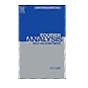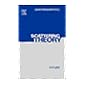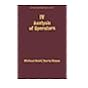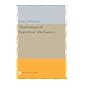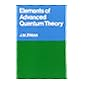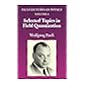530-197x Fourier Analysis, Self-Adjointness (Methods of Modern Mathematical Physics, Vol. 2). 530-197x Scattering Theory (Methods of Modern Mathematical Physics, Vol. 3). 530-197x IV: Analysis of Operators, Volume 4 (Methods of Modern Mathematical Physics). 530-197x Mathematical statistical mechanics 530-197x Elements of advanced quantum theory, 530-197x Selected topics in field quantization / 530-197x Statistical mechanics /

Bibliography: pages 263-274.

## Other editions of this work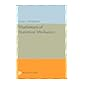Mathematical statistical mechanics / by Thompson, Colin J.,Mathematical statistical mechanics / by Thompson, Colin J.,Mathematical statistical mechanics / by Thompson, Colin J.,Mathematical statistical mechanics / by Thompson, Colin J.,
Share